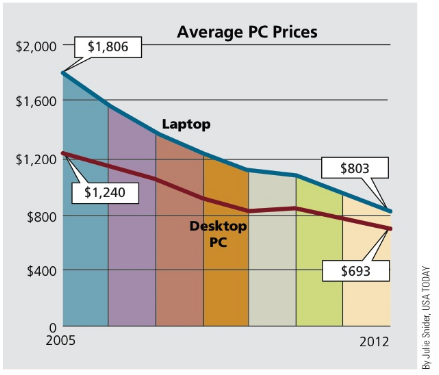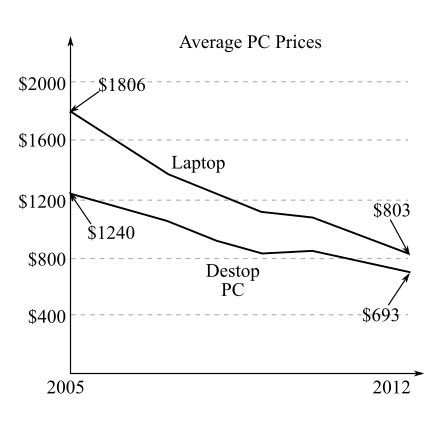Chapter 1.III, Problem 29REContemporary Mathematics for Busin...

8th Edition
Robert Brechner + 1 other
ISBN: 9781305585447

Solutions

Chapter
SectionContemporary Mathematics for Busin...

8th Edition
Robert Brechner + 1 other
ISBN: 9781305585447
Textbook Problem

As the IT manager for FastNet Enterprises, you have maintained records of the average prices you’ve paid for PCs over the years, and you are reviewing your records over a particularly interesting period in your company’s history. In 2005, you purchased 12 laptop computers and 15 desktop computers for your office staff. Using the graph Average PC Prices, answer the following:a. What was the total amount of the purchase for these computers in 2005?b. In 2012, you replaced all of the computers with new ones. What was the total amount of the purchase for these computers?c. In total, how much did you save in 2012 over 2005 because of falling computer prices?(a)

To determine

To calculate: The total amount of purchase of 12 laptop computers and 15 desktop computers in 2005 by the use of the graph,Explanation

Given Information:

The purchase consist of 12 laptop computers and 15 desktop computers.

The provided graph is,

Formula used:

Steps for multiplying whole numbers.

Step 1: Write down the factors such that the place values line up in columns.

Step 2: Multiply every digit of the multiplier with the multiplicand, starting with the units. Each multiplication gives a partial product whose unit digit appears under the same column that is the corresponding digit of the multiplier.

Step 3: Now in each column, add the digits of the partial products, starting with the unit’s column on the right.

Calculation:

Consider the given data,

The purchase consists of 12 laptop computers and 15 desktop computers.

According to the graph, the average price of desktop pc in 2005 is $1,240. The average price of laptop in 2005 is$1,806.

So, Total price of desktop computers will be,

1240×         15               ¯

To find value of multiplication,

Multiply the multiplier ‘5’ with the multiplicand:

1240×         15     18600 ¯

Multiply the multiplier ‘1’ with the multiplicand and write the partial product under the corresponding column:

1240×         15     18600     1240 ¯

Add the partial products,

1240×         15       6200     1240     18600 ¯ ¯

Therefore, total price of desktop computers is \$18,600

(b)

To determine

To calculate: The total amount of purchase if all the computers were replaced by the new ones in 2012 by the use of graph,(c)

To determine

To calculate: The total amount of money that is saved due to the falling computer prices in 2012 over 2005.

Still sussing out bartleby?

Check out a sample textbook solution.

See a sample solution

The Solution to Your Study Problems

Bartleby provides explanations to thousands of textbook problems written by our experts, many with advanced degrees!

Get Started

|2x 3| 5

Applied Calculus for the Managerial, Life, and Social Sciences: A Brief Approach

Find y and y. y=1(1+tanx)2

Single Variable Calculus: Early Transcendentals

Let and g(x) = x + 3. Then (g ∘ h)(x) = ______.

Study Guide for Stewart's Single Variable Calculus: Early Transcendentals, 8th